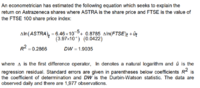# F-Test Probability

#### iiownz

##### New member
Hi guys!

I've been trying to solve this exercise for a long time. I know that is not that dificult.

However i cant remember how to do it.

Ho = 0
H1 dif 0

1977 observations.

I was wondering that to calculate the F-test i should have the standard error for the Y variable, but i dont have.
Also, i was expecting to do sd1/sd2 to get my F and make a comparison using the F table, but i am not sure if this is right.Calculate the F-test statistic for this equation and use it to perform a test for the null hypothesis that the slope coefficient is equal to zero

#### iiownz

##### New member
I found this relation on the internet. Where does it come from? I have never heard about it.

F= (R^2/(p-1))/((1-R^2)/(n-p))

Should i use p = 2 because there are two variables - Astra and FTSE? or because there are 2 variables (slope coeficient + linear coeficient)?

up!